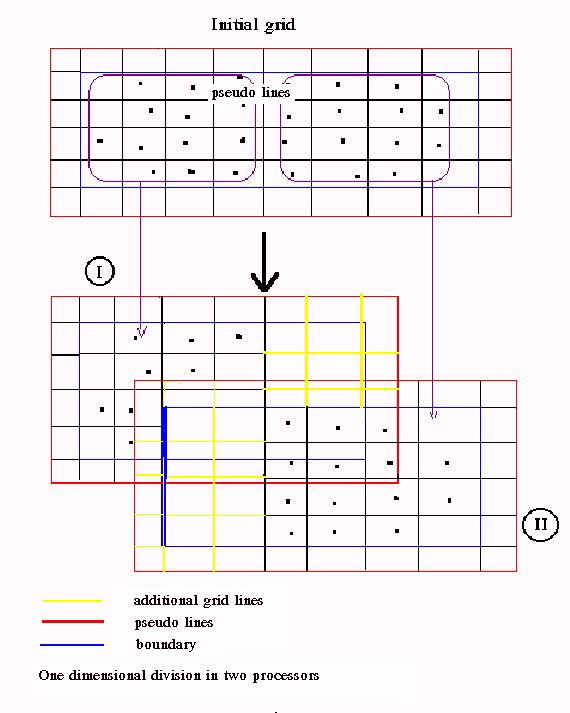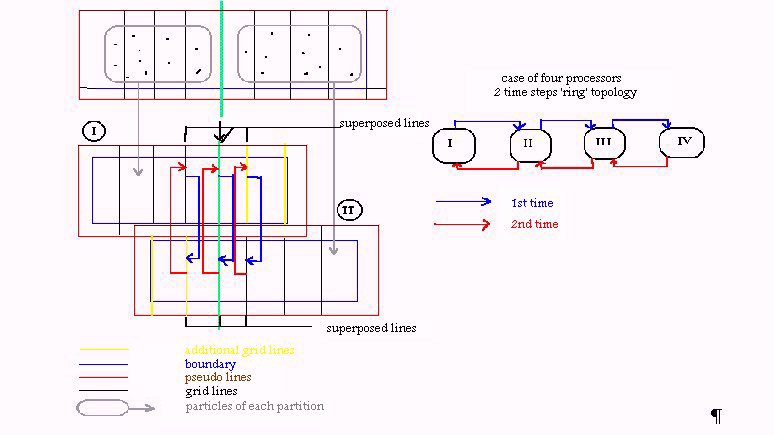# PARTICLE MESH METHODS FOR DISTRIBUTED MEMORY MACHINES

D. G. Triantos, K. Pothou

National Technical University of Athens, Fluids Section,

PO Box 64070, 15710 Zografou, Athens, Greece

tel.:(+)301-7721096, fax:(+)301-7721057, e_mail: aiolos@fluid.mech.ntua.gr

INTRODUCTION

## Introduction

In order to approximate the velocity field in C.F.D. applications using Langragian approaches, pointwize methods using particles have been introduced. These methods are sufficiently accurate inside their limitations, due to the existence of analytical expressions for the velocity field in most cases. However the computational cost of the particle method is prohibitive for applications which need large numbers of particles, for example applications of unsteady phenomena. In order to overcame this difficulty Particle Mesh methods have been introduced. These methods lead to a significant time gain for slightly less accuracy. However the study of complex unsteady phenomena demand large amounts of computational time even with the use of Particle Mesh methods.

Today 's availability of concurrent computers show a way to brake through the problem. In this work we shall try to present a concurrent Particle Mesh method suitable for distributed memory machines. In our attempt to develop a code that will run efficiently without any changes in a wide range of parallel computers as well as in a network of serial computers under a message passing platform we used the BLACS interface .

## Particle Mesh algorithm and parallelization strategy

Suppose that we have a set of particles of total number NP, which are determined in the computational space Vp, by their position Xi={ xi,yi,zi} i=1...NP, and their intensity G={gxi,gyi,gzi} i=1..NP. In order to calculate the velocity and the deformation of each particle with a Particle Mesh method the next steps should be followed.

I. A rectangular mesh that contains all the particles, should be created inside the computational space. The boundaries of this mesh should be sufficiently far from the particles. Let nx, ny, nz be the number of nodes at each direction.

II. The vector potential values at each node of the boundary of the mesh should be calculated analytically as the superposition of the contribution of each particle {Fig 1}.

III. An extrapolation method should be introduce in order to evaluate the discrete intensity field at the nodes of the mesh. The extrapolation method that will be used should interrelate the intensity of each particle to a number of neighbour nodes. The number of the nodes and the scheme of the extrapolation could lead to the addition of an extra grid line outside the boundary of the mesh and it is related to the accuracy of the Particle Mesh method. Figure 2 shows an extrapolation of a particle to the 9 neighbour nodes

IV. In this step a method (usually Fourier transform ) that solves the Poisson equation that relates the potential and the vorticity is used in order to evaluate the potential field on the meshFigure 1

V. Since the potential field is evaluated the velocity and the deformation field on the mesh can be calculated. An interpolation procedure ( exact opposite of the extrapolation procedure that has been used ) is performed in order to interrelate the mesh values of the velocity and the deformation to the particles.Figure 2

In order to parallelize this algorithm for a distributed memory machine the following strategy was implemented.

Suppose that NPR is the number of processors that can be used. The first step is to divide the mesh so that each processor takes a part of it and the corresponding particles that exist in that part. The division should be in a way that all processors take equal nodes and equal particles as possible, so all processors carry the same load. Suppose that the mesh is rectangular the division could be according the {Fig 3} in one or in two dimensions. The optimum way is related with the number of the available processors.

In the partition of the grid corresponding to processor, few grid lines (usually two) should be added at each edge at the direction of the division so that the boundary of each partition is sufficiently far from the included particles {Fig 4}. These additional grid lines are necessary for the accuracy of the results but also give an additional overhead. If the extrapolation method we used demand an extra grid line (pseudo line) out of the border this should be added too.Figure 3Figure 4

Suppose that each processor has each computational space which is consisted by the corresponding partition of the grid and the included particles (positions and intensities ). The next step is the calculation of the boundary conditions. The calculations are performed as in the serial algorithm with the addition of broadcasting the particles (positions and intensities ) of each processor to all the others in order to succeed the superposition of the contribution of all the particles. In order to achieve load balance the procedure shown at {Fig 5} was implemented.Figure 5

The third step, the extrapolation procedure can be performed identical to its serial form by each processor on it 's corresponding computational space. During this procedure discrete fields of intensity have been created on the mesh for each processor. The values of these fields which are on nodes neighbour to other processor partition need to be superposed in order to get the influence of the particles that belong in a neighbour partition. A 'ring' topology of two steps has been used in order to communicate the values that should be added. The procedure is presented in Figure 6.Figure 6

In the fourth step of solution the serial code was used since Fourier transform methods lead to tridiagonal systems which are very fast to be resolved. At the end of this step communication of some values of the just estimated discrete potential field has to be implemented, since in the next step the calculation of the velocity and the deformation field is performed with a finite difference's method. The same 'ring' topology with two steps as in the previous step is used as it is described in Figure 7.Figure 7

In the fifth step again the serial code is used on each partition without the need of any communication. With the end of the fifth step at each partition of the initial mesh corresponding to each processor the velocities and deformations of the particles are calculated.

In order to estimate the theoretical expected speed up the following should be kept onThe number of processors is restricted by the fact that the number of the grid nodes at each direction of each partition of the grid should be an odd number. This is due to the use of the Fourier transform.Each partition at each direction shouldn't have less than 3 cells.The overhead of the presented algorithm is due to the communication between the processors, due to the calculation of additional boundary conditions, since with the division of the initial mesh new boundaries are created and finally due to the addition of grid lines at the edge of each partitions as its described above.

Supposing that the overhead due to communication and the boundary condition is zero the speed up could be estimated as follows:

One dimension division of the initial grid:

Suppose at the x direction the total number of nodes is nx ( pseudo nodes included ) and Np the available processors such as: ( nx - 3 ) / NP = ts where ts is an odd number. Suppose that 2 additional lines at each edge are used. Then the theoretical expected speed up is: ( nx - 3 ) / ( ts + 4 ) = TES

Two dimension division of the initial grid:

Using an analogue procedure where NP = NPx * NPy and the additional lines are 2 the following formula is developed:The expected theoretical speedup is quit close to the real speed up especially when the number of the particles is small and it also points out the optimum division.

## Results

In order to test efficiency of the parallel version the following test was implemented. In a three dimensional grid ( nx = 43, ny = 43, nz = 43 ), 76 vortex particles were distributed around a square, with total circulation equal to two. The particles vorticity was an exponential function of the side of the square. The square was set at the centre of the grid. In Figure 8 the Uz component of the velocity is presented as a function of x on the two sides of the square. Analytical results with a Biot Savar method are also presented on the same figure.

The same three dimensional grid of ( nx = 43, ny = 43, nz = 43 ) was used in order to test speedups for various numbers of particles. In this case runs were performed for a wide range of vortex particles (from 10 up to 100000 ) of total circulation 1, distributed on a circle of radius 1. Times in CPU seconds of the serial and the parallel version are presented in Figure 9 and the corresponding speedup in Figure 10.

For both cases the mesh was deviated one dimensionally on 10 processors and the theoretical expected speed up according the given formula is 5.Figure 8Figure 9Figure 10

## References

 J. J. Dongarra, R. C. Whaley "A User' s Guide to the BLACS v 1.0" LAPACK working note 94, June 7, 1995